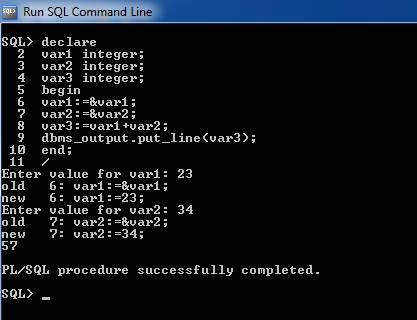# PL/SQL Program To Add Two Numbers

In the previous tutorial we have learnt much about PL/SQL variables, operators, data types and other such fundamental topics. Let us get into real programming now onwards.

For starters, let us look at a PL/SQL program to add two numbers or integers and fetching the result into a third variable.

This program takes two inputs one for each variable and adds the result to a third variable and prints it.

## PL/SQL Program To Add Two Numbers

OutputSince, we need variables in this program code so we need to declare them in the declaration section. In the begin section, we need to initialize and take input from the user. All the logical and mathematical calculations for this program logic need to be established here.

Var1:=&var1;
This statement assigns the value that the user enters for the variable. Similarly, we have taken input for next variable.

Var3:=var1+var2
This statement is used to assign the calculated values of Var1 and Var2 into the third variable.

Dbms_output.put_line()
The statement takes a parameter which can be printed onto the console screen.

Note: For this statement to work properly, it is sometimes necessary to use a particular statement as mentioned below:

When you start the SQL Command Prompt/Terminal, just type:

Set serveroutput on;

This statement is used to activate the working of print statement on the console screen. This will help to get the proper output onto the screen/terminal as expected.

Finally, the End statement is used to terminate the code.

### 16 thoughts on “PL/SQL Program To Add Two Numbers”

1.thanks!!!!!!!!

2.hii…thank you it was very helpful
can you explain how to find largest of 2/3 numbers?

1.declare
var1 number;
var2 number;
begin
var1:=&var1;
var2:=&var2;
if var1<var2 then
dbms_output.put_line('var2 is greater');
else
dbms_output.put_line('var1 is greater');
end if;
end;
/

3.SUMIT KUMAR GUPTA

Thank u sir for sharing this program

4.thank you sir for sharing this program……

5.not working in oracle12g

6.Ronikarajalakshmi

hello, please put on algorithm steps from program codings in pL/SQL

7.hello!!! pls update some crucial informations and some programs in below email.

8.why are you using ( := ) this operator ?
please explain now my comment …

1.bcoz its the way we assign value to variable.

9.how to create simple calculator in oracle pl sql developer

10.i m a student and i wanna know how to create simple calculator in pl sql developer please share query .. or recommend the exact website where i can find

11.how to write a stored procedure to insert the employee details in a table
conditions: write it for one month,30 days will be displayed,holidays dates will be skipped.

12.Write a plsql code, which takes two numbers and operator as input from user
and then apply that operator on given number. (use arithmetic operator +,-, /,*).

13.Thank you sir

14.How to add 2 numbers without using + operator in PL/SQL